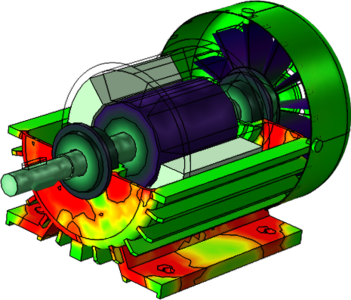# Galleria delle Applicazioni

## Modeling Vibration in an Induction Motor

Application ID: 47871

In the Vibration in an Induction Motor tutorial model, eddy currents are induced in the rotor by time-harmonic currents in the stator windings and rotation of the rotor. Induced currents in the rotor interact with the magnetic field that is produced by the coils to generate the driving torque on the rotor. The air gap between the rotor and stator is asymmetric, resulting in vibrations in the motor.

The electromagnetic simulation is modeled in 2D, whereas the multibody dynamics simulation is modeled in 3D. The rotational torque is calculated as a function of time. The torque is then used in the multibody dynamics model in order to compute the rotor speed, accounting for inertial effects. Due to resistive losses and loading torque on the shaft, the rotor will not revolve as fast as the stator windings, so an angular slip will occur.

Find out more about this example in a related blog post: "Analyzing the Structural Integrity of an Induction Motor with Simulation"This model example illustrates applications of this type that would nominally be built using the following products: